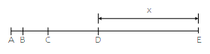# Problem 45536. Distance Between Points

Being n the number of points (If n = 3 there would be 3 points: A, B and C). The distance between each pair of points increases progressively twice, starting with points A and B.Given n as an integer greater than 1 and x as the distance between the last pair of points (D and E in figure).

Find the distance between the first and last points y.

### Solution Stats

56.76% Correct | 43.24% Incorrect
Last Solution submitted on Aug 29, 2023

### Community Treasure Hunt

Find the treasures in MATLAB Central and discover how the community can help you!

Start Hunting!